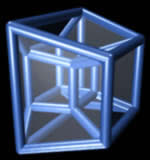# Dimensions

In Geometry we can have different dimensions.

The number of dimensions is how many values are needed to locate points on a shape.## Point, Line, Plane and Solid

A Point has no dimensions, only position
A Line is one-dimensional
A Plane is two dimensional (2D)
A Solid is three-dimensional (3D)A point really has no size at all! But we show them as dots so we can see where they are.Now let's allow the point to move in one direction. We get a line.

We need just one value to find a point on that line. So we have one dimension. A line is one-dimensional.Now lets allow the point to move in a different direction. And we get a plane. We need two values to find a point on that plane. So we have two dimensions, or "2D".

Circles, triangles, squares and more are plane shapes.Now we let that point move in another completely different direction and we have three dimensions.

Spheres, cubes, cylinders and more are 3-dimensional or "3D". We also call them solid shapes.

The world we live in is 3D.In mathematics we can have more dimensions (such as this 4D Tessaract), but they are hard to draw!

10307, 10308, 10309, 10310, 10311, 10312, 10313, 10314, 10315, 10316